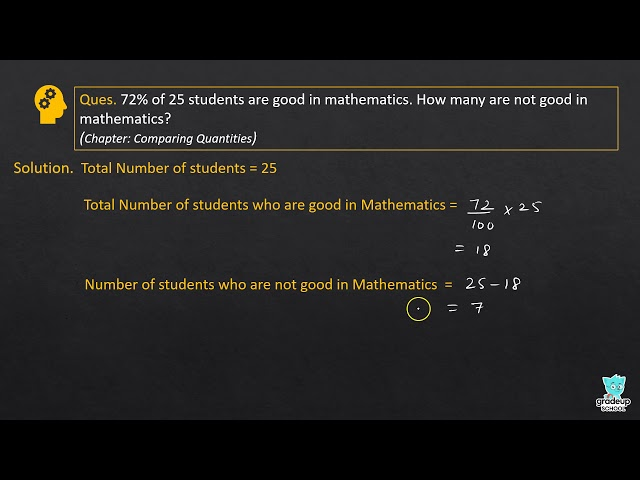# NCERT Solutions for Class 8 Maths Chapter 8 - Comparing Quantities

Share

NCERT Mathematics Solutions for Class 8 Chapter 8 - Comparing Quantities prepared by experienced subject mentors of Goprep in order to help students in preparing for the exam. These NCERT Solutions are crafted after considering the latest CBSE syllabus in order to help you to understand the concepts and solve the questions of the textbook in an efficient way. Further, accessing these Solutions is easy and without incurring any cost!

Take your calculation skills ahead with these Solutions of Chapter 8 which helps students to determine the Quantities, Percentage, Interest and other Numbers. Further, you will learn Ratios and Percentages, Finding the Increase or Decrease percent, Finding Discounts, Finding Cost Price/Selling Price, Compound Interest, Sales Tax/ Service Tax, Deducing a Formula for Compound Interest, Rate Compounded Yearly or Half Yearly, Applications of Compound Interest Formula etc by using these NCERT Chapter-wise Solutions.

## NCERT Solutions for Class 8 Maths Chapter 8 - Comparing Quantities

Exercises 8.1
• Exercises 8.1
• Exercises 8.2
• Exercises 8.3
Class 8th|NCERT - MathematicsChapter 8 - Comparing QuantitiesNCERT - MathematicsClass 8th , NCERT
 Chapter 1 - Rational Numbers Chapter 2 - Linear Equations in One Variable Chapter 3 - Understanding Quadrilaterals Chapter 4 - Practical Geometry Chapter 5 - Data Handling Chapter 6 - Squares and Square Roots Chapter 7 - Cubes and Cube Roots Chapter 8 - Comparing Quantities Chapter 9 - Algebraic Expressions and Identities Chapter 10 - Visualising Solid Shapes Chapter 11 - Mensuration Chapter 12 - Exponents and Powers Chapter 13 - Direct and Inverse Proportions Chapter 14 - Factorisation Chapter 15 - Introduction to Graphs Chapter 16 - Playing with Numbers